# Disaster Watcher¶

## Disaster Identification using Tweeter Data and Deep Learning¶

The sole purpose of this notebook is to presents and outlines the steps that was taken to train the model.

Due to the large run time, the model training section was not run in this notebook.The actual production jupyter notebook which was trained using google Colab can be found in the github repo.

## 1. Introduction¶

Social media is increasingly being used to broadcast useful information during local crisis situations(e.g. hurricanes, earthquakes, explosions, bombings,etc).Identifying disaster related information in social media is challenging due to the low signal-to-noise ratio.In this work we will use NLP to address this challenge.

Some of the tweets sent from mobile devices can be geotagged containing the precise location coordinates. However, only about 1% to 3% of all tweets are geotagged.Identifying the disaster related tweets along with their is highly valuable to for the first responders in the disaster and crisis situations. In this project we fist. identify the disaster related tweets from a deep learning model and then use Named Entity Recognition library to identify and map the location of the data.

## 2. Data¶

The natural disaster events generally generate a massive and disperse reaction in social media channels.Users usually express their thoughts and actions taken before, during, and after the storm. We used the classified crisis related tweets collection from the CrisisLex.org which is a repository of crisis-related social media data. We used the CrisisLexT6 dataset which includes Tweets from 6 crises, labeled by relatedness.

• Contents: ~60K tweets posted during 6 crisis events in 2012 and 2013.
• Labels: ~60,000 tweets (10,000 in each collection) were labeled by crowdsourcing workers according to relatedness (as "on-topic", or "off-topic").

The data from the following crisis events were used in this analysis :

• Flood
• Earthquake
• Hurricane
• Explosion
• Bombing

## 3. Preprocessing¶

The preprocessing of the text data is an essential step in any NLP and text classification analysis and machine learning algorithms.[]The objective of this step is to clean noise those are less relevant to find the sentiment of tweets such as punctuation, special characters, numbers, and terms which don’t carry much weightage in context to the text.[] Lets first import the required packages.

In :
%%capture
%matplotlib inline

import os
import numpy as np
import pandas as pd
import matplotlib.pyplot as plt
import seaborn as sns
import tensorflow as tf
from sklearn.base import TransformerMixin ,BaseEstimator
from sklearn.pipeline import Pipeline
from sklearn.preprocessing import LabelEncoder
from sklearn.model_selection import train_test_split
from tensorflow.keras.utils import to_categorical
from tensorflow.keras.models import Sequential ,model_from_json
from tensorflow.keras.layers import Embedding,Dense,Dropout ,GlobalMaxPool1D

from IPython.display import clear_output
from tensorflow.keras.wrappers.scikit_learn import KerasClassifier
from sklearn.model_selection import RandomizedSearchCV


The data are stored in 11 csv formated files.We first load this data and then save it into a single combined file for further analysis.The name of the file is the same as the name of the crisis.The Tweets in each files has been labeled as "on-topic" or "off-topic" and do not contains information about the type of the crisis.However, the the type of the crisis is represented in the file name.We will use these file name to assign proper labels to each category. First lets load the data and have quick look at it.

We will be using the Pipeline from Sklean library to streamline the preprocessing routine. As a result the the classes in the analysis should be compatible with the pipeline arcitecture.

In :
COMBINDED_DATASET='combined.csv'
DATA_DIRECTORY='../datasets'
class DatasetExtractor(BaseEstimator,TransformerMixin):
"""Extractor class that loads multiple Tweet files and creates a single unified file."""

def transform(self,X,y=None):

combined_file_path=f'{DATA_DIRECTORY}/{COMBINDED_DATASET}'
if os.path.isfile(combined_file_path):
combined_dataset.to_csv(combined_file_path)
return combined_dataset

"""Loads multiple disaster related tweet file and returns a Single Pandas data frame"""
combined_dataset=pd.DataFrame()
for file_name in os.listdir(path=DATA_DIRECTORY):
category=self.extract_category_name(file_name)
df['category']= category
combined_dataset=combined_dataset.append(df,ignore_index = True)
return  combined_dataset

def extract_category_name(self,file_name):
"""Helper method that extracts the Disaster Category from the file name"""
category=file_name.split('.')
if '_' in category:
category=category.split('_')
return category


For the purpose of demonstration We load each part of the pipeline to provide explanation and explanation about each part separately .Ultimately we chain all of these methods into a pipeline for the final modeling. Lets load the data and see how it looks like:

In :
dataset=DatasetExtractor().transform(None)

File Exists.Reloaded.

Out:
tweet id tweet label category
0 '348351442404376578' @Jay1972Jay Nope. Mid 80's. It's off Metallica... off-topic floods
1 '348167215536803841' Nothing like a :16 second downpour to give us ... off-topic floods
2 '348644655786778624' @NelsonTagoona so glad that you missed the flo... on-topic floods
3 '350519668815036416' Party hard , suns down , still warm , lovin li... off-topic floods
4 '351446519733432320' @Exclusionzone if you compare yourself to wate... off-topic floods

As mentioned, the data consistes of the following features:

• tweet id
• tweet
• label
• category

The category feature was inferred from the file name and added to the data during the loading. The labels were assigned using human labels for each crisis.We will only use the "on-topic" tweets from each category.All the "off-topic" tweets would be combined and would be classified as the "unrelated".

## 3.2 Data Cleaning¶

#### 3.3.1 Text cleaning¶

Tweets can contain many different kind of noise that can negatively affect the performance of the machine learning algorithms . We need to carefully get rid of them. We will use the of regular expressions and replace functionality in Pandas to remove the unwanted noise in the data.

#### 3.3.1 Re-Tweets:¶

They add no real value to the data and can sometimes lead to overfitting

#### 3.3.2 URL's¶

They do not deliver any predictive power, The sentiment of a tweet can not be judged by reading an URL. In the worst case scenario they might lead to overfitting.

df['tweet']=df['tweet'].str.replace('http\S+', '',regex=True)

#### 3.3.3 Symbols¶

Hashtags, commas, points and and all kind of punctuation symbols are removed.

df['tweet']=df['tweet'].str.replace('[^a-zA-Z\s]', '',regex=True)

#### 3.3.3 White Spaces¶

We also get ride of any additional white spaces in the texts that might be created due to the previous steps.

df['tweet']=df['tweet'].str.strip()

df['tweet']=df['tweet'].str.replace('\s+', '',regex=True)

#### 3.3.4 Lower case¶

All texts are transformed to lowercase.

#### 3.3.4 Location Names¶

The names of the location which disaster happened were repeated in so many tweets.We want to prevent the model from associating these location names with the crisis and as a result we remove the most frequent ones from the Tweets. The follwing list of words were removed from the Tweets:

In :
STOP_WORDS=["Boston", "Oklahoma","Texas","Nepal","California","Calgary","Chile","Alberta","Pakistan" ,"WestTX","Canada","yycflood","USA","'S",]
class DatasetCleaner(BaseEstimator,TransformerMixin):
"""Removes Redundent features and rows with missing values"""
def transform(self,X,y=None):
columns=X.columns.tolist()
X.columns=[column.strip() for column in columns]
X=X.drop('tweet id',axis=1)
X=X.dropna()
X['tweet']=X['tweet'].str.replace('@', '')
X['tweet']=X['tweet'].str.replace('#', '')
X['tweet']=X['tweet'].str.replace('.', '')
X['tweet']=X['tweet'].str.replace(',', '')
X['tweet']=X['tweet'].str.replace('http\S+', '',regex=True)
X['tweet']=X['tweet'].str.replace('@\w+', '',regex=True)
X['tweet']=X['tweet'].str.replace('\s+', '',regex=True)
X['tweet']=X['tweet'].str.strip()
X['tweet']=X['tweet'].str.lower()
for word in STOP_WORDS:
word=word.lower()
X['tweet']=X['tweet'].str.replace(word, '')
return X

In :
dataset_cleaned=DatasetCleaner().transform(dataset)

Out:
tweet label category
0 jay1972jaynopemid80itoffmetallica2ndalbumridet... off-topic floods
1 nothinglikea:16seconddownpourtogiveussomemuchn... off-topic floods
3 partyhardsunsdownstillwarmlovinlifesmileharddo... off-topic floods
4 exclusionzoneifyoucompareyourselftowaterdoesth... off-topic floods

## 3.3 Re-Sampling¶

Lets take a look to see how many Tweets do we have in each category regardless of being on or off topic.We want to make sure the number of tweets in each category are in the same order and we have a balanced dataset.We would also shuffle the tweets to make sure that the tweets have no particular order. Lets first see how many tweets we have in each category.This would be total number of tweets.Each file has on-topic and off-topic tweets which is the way they have been labeled by human labelers.

In :
Crisis=pd.DataFrame(dataset['category'].value_counts())
Crisis.reset_index(inplace=True)
Crisis.rename(columns={'index':'Crisis',"category":'Tweet Count'} ,inplace=True)
Crisis

Out:
Crisis Tweet Count
0 floods 20064
1 bombing 10012
2 hurricane 10008
3 explosion 10006
5 earthquake 9057
In :
f,ax =plt.subplots(figsize=(15,7))
sns.barplot(x='Crisis',y='Tweet Count',data=Crisis ,palette=sns.light_palette((210, 90, 60),10, input="husl" ,reverse=True),ax=ax)
ax.set_xlabel(' ')

Out:
Text(0.5, 0, ' ')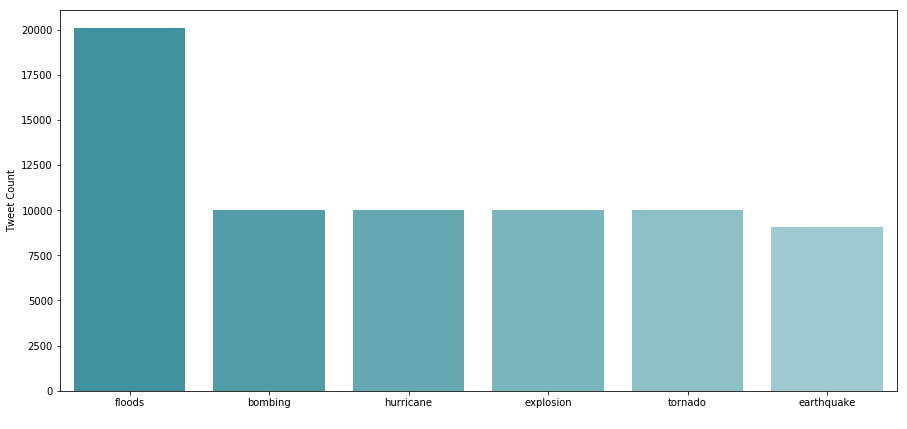#### 3.3.1 All Tweets ( On-Topic and off-Topic ) for each category¶

As you can see we have roughly about 10,000 tweets for each crisis, except floods.As a next step lets see how many related (on-topic) Tweets we have in each category.This is more important since we are only using the on-topic Tweets from each category during the classification.

In :
dataset['label_full']=dataset['label']+'_'+dataset['category']
Crisis_topics=pd.DataFrame(dataset['label_full'].value_counts())
Crisis_topics.drop('On-topic_earthquake',axis=0,inplace=True)
Crisis_topics.reset_index(inplace=True)
Crisis_topics.rename(columns={'index':'Crisis',"label_full":'Tweet Count'} ,inplace=True)

Crisis_topics

Out:
Crisis Tweet Count
0 on-topic_floods 10603
1 off-topic_floods 9461
2 on-topic_hurricane 6138
3 on-topic_bombing 5648
4 on-topic_explosion 5246
7 off-topic_explosion 4760
8 on-topic_earthquake 4580
9 Off-topic_earthquake 4475
10 off-topic_bombing 4364
11 off-topic_hurricane 3870
In :
f,ax =plt.subplots(figsize=(15,7))
sns.barplot(y='Crisis',x='Tweet Count',data=Crisis_topics ,palette=sns.light_palette((210, 90, 60),20, input="husl" ,reverse=True),ax=ax)
ax.set_xlabel(' ')
ax.set_title('Numer of on-topic and off-topic Tweets in each crisis Category ')

Out:
Text(0.5, 1.0, 'Numer of on-topic and off-topic Tweets in each crisis Category ')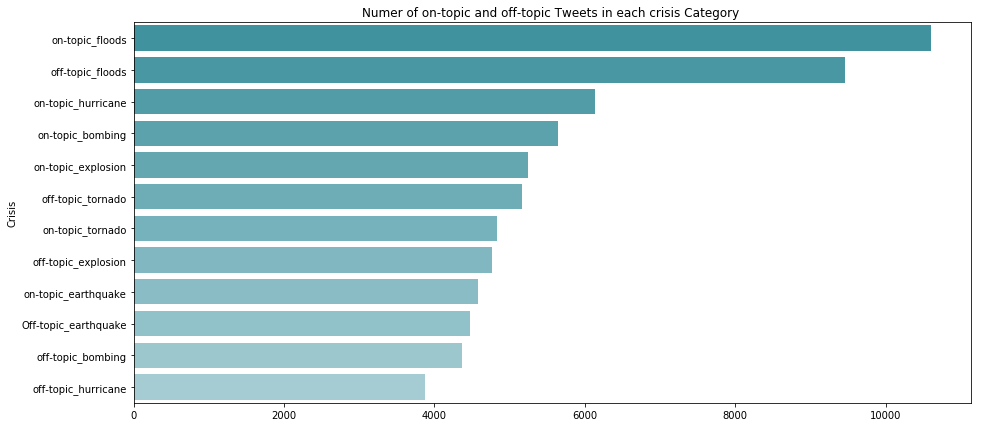#### 3.3.2 On-Topic Tweets¶

Lets take a look at only the on topic Tweets in each Category: We can see that labels are balanced. We have about 5000 on-topic Tweets in each category(except flood).

In :
Crisis_topics_on_topic= Crisis_topics[Crisis_topics['Crisis'].str.contains("on-topic")]
Crisis_topics_on_topic['Tweet_pct']=Crisis_topics_on_topic['Tweet Count']*100/Crisis_topics_on_topic['Tweet Count'].sum()
Crisis_topics_on_topic

/home/adminn/.local/lib/python3.6/site-packages/ipykernel_launcher.py:2: SettingWithCopyWarning:
A value is trying to be set on a copy of a slice from a DataFrame.
Try using .loc[row_indexer,col_indexer] = value instead

See the caveats in the documentation: http://pandas.pydata.org/pandas-docs/stable/indexing.html#indexing-view-versus-copy


Out:
Crisis Tweet Count Tweet_pct
0 on-topic_floods 10603 28.624264
2 on-topic_hurricane 6138 16.570380
3 on-topic_bombing 5648 15.247557
4 on-topic_explosion 5246 14.162302
8 on-topic_earthquake 4580 12.364343
In :
f,ax =plt.subplots(figsize=(15,7))
sns.barplot(x='Crisis',y='Tweet_pct',data=Crisis_topics_on_topic ,palette=sns.light_palette((216, 100, 40), input="husl" ,reverse=True),ax=ax)
ax.set_xlabel(' ')
ax.set_ylabel('Percentage of on-topic Tweets in Each Category')

Out:
Text(0, 0.5, 'Percentage of on-topic Tweets in Each Category')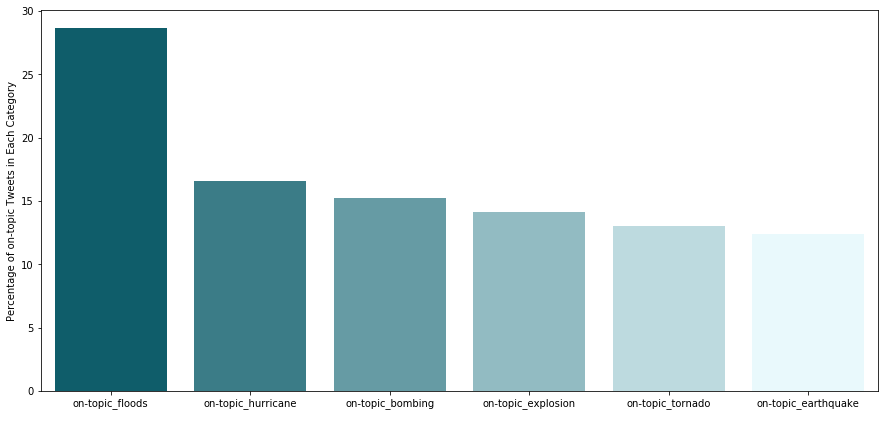#### 3.3.3 Off-Topic Tweets¶

To avoid overfitting we also use a random set of off-topic tweets from each of the categories.We label all these tweets as unrelated. Using these additional label would let the model learn to better distinguish between the related and unrelated tweets for each category.

In :
Crisis_topics_off_topic= Crisis_topics[~Crisis_topics['Crisis'].str.contains("on-topic")]
total_off_topic_tweets=Crisis_topics_off_topic['Tweet Count'].sum()
print("Total Number of 'Off-Topic' Tweets",total_off_topic_tweets)

Total Number of 'Off-Topic' Tweets 32095

Out:
Crisis Tweet Count
1 off-topic_floods 9461
7 off-topic_explosion 4760
9 Off-topic_earthquake 4475
10 off-topic_bombing 4364
##### 3.3.3.1 Imbalanced Data¶

Imbalanced data generally refers to an issue with classification problems where the classes are not represented equally.In our case, since each category has it own off-topic tweets, the total number of off-topic tweets from all of the categories would be way higher than the on-topic tweets in each category.This would make our database highly imbalanced.

Lets plot the total number of off-topic tweets along with the on-topic tweets.Note that the "off-topic" would also be one of our prediction categories, as a result, this category should also have the same number of tweets (roughly) as the other categories.

Lets label all of these tweets with an unrelated label.

In :
Crisis_topics_off_topic['Crisis']='unrelated'
Crisis_topics_off_topic_g=Crisis_topics_off_topic.groupby(by='Crisis').sum()
Crisis_topics_off_topic_g.reset_index(inplace=True)
all_topics =Crisis_topics_off_topic_g.append(Crisis_topics_on_topic[['Crisis','Tweet Count']])
all_topics

/home/adminn/.local/lib/python3.6/site-packages/ipykernel_launcher.py:1: SettingWithCopyWarning:
A value is trying to be set on a copy of a slice from a DataFrame.
Try using .loc[row_indexer,col_indexer] = value instead

See the caveats in the documentation: http://pandas.pydata.org/pandas-docs/stable/indexing.html#indexing-view-versus-copy
"""Entry point for launching an IPython kernel.

Out:
Crisis Tweet Count
0 unrelated 32095
0 on-topic_floods 10603
2 on-topic_hurricane 6138
3 on-topic_bombing 5648
4 on-topic_explosion 5246
8 on-topic_earthquake 4580
In :
f,ax =plt.subplots(figsize=(15,7))
blues=sns.light_palette((216, 100, 40),all_topics.shape, input="husl" ,reverse=True)
blues=sns.color_palette("RdBu", 10)
sns.barplot(x='Crisis',y='Tweet Count',data=all_topics ,palette=blues, ax=ax)
ax.set_xlabel(' ')
ax.set_ylabel('Number of Tweets')
ax.set_title( 'Imbalanced Dataset: Total Number of Tweets in each category')

Out:
Text(0.5, 1.0, 'Imbalanced Dataset: Total Number of Tweets in each category')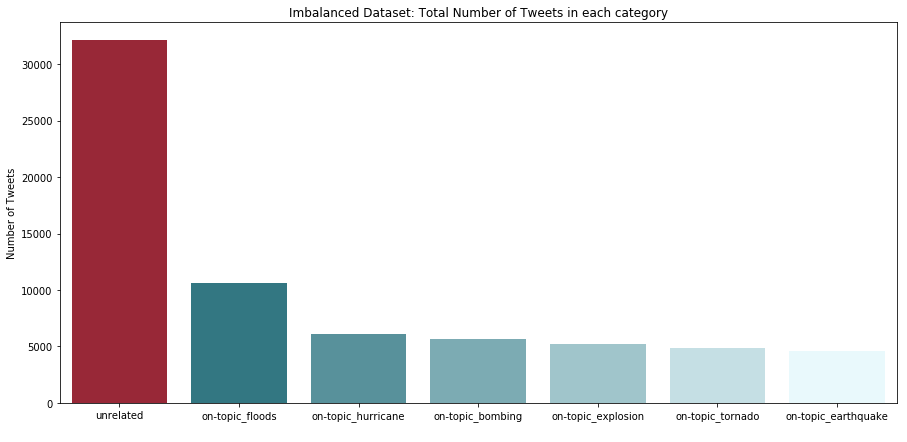##### 3.3.3.2 balancing the Data¶

As we can see the number of unrelated tweets are way higher than the actual on topic tweets.To solve the problem, we resample a subset of these these unrelated Tweets.The total number that we re-sample from these unrelated tweets would be equal to the average number of all tweets in each dataset.

In :
class DistributionValidSampler(BaseEstimator,TransformerMixin):
"""Samples the (related and random ) tweets with equal proportion"""
def __init__(self,unrelated_size=None ,ignore_unrelated_proportion=True):
self._unrelated_size=unrelated_size
self._ignore_unrelated_proportion=ignore_unrelated_proportion

def transform(self,X,y=None):
#Shuffle tweets
X_=X.sample(frac=1).reset_index(drop=True)
X_=self._label_categories(X_)
related,unrelated =self._equal_split(X_)
X_=self._merge(related,unrelated)
X_=X_.drop('category',axis=1)
return X_

def _label_categories(self,X):
"""Assings the category name to on-topic tweets and unrelated to off-topic tweets in
each category
"""
if self._ignore_unrelated_proportion:
X['label']=X.apply(lambda row: row['category'] if 'on-topic' in row['label'] else 'unrelated',axis=1 )
else:
X['label']=X.apply(lambda row: row['category'] if 'on-topic' in row['label'] else 'unrelated_'+row['category'],axis=1 )
return X

def _equal_split(self,X):
"""Splits the dataseta into related and unrelated tweets.
This ensures that the number of unrelated tweets are not too high and
is in reasonable range.
"""
related=X[X['label'].str.contains('unrelated')==False]
unrelated=X[X['label'].str.contains('unrelated')]
ave_tweets=self._average_tweet_per_category(X)
unrelated=self._slice(unrelated,size=self._unrelated_size ,ave_size=ave_tweets)
return related,unrelated

def _merge(self,X1,X2):
"""Merges the dataframes toghether"""
X=pd.DataFrame()
X=X.append(X1)
X=X.append(X2)
return X

def _slice(self,X, size ,ave_size):
"""Extracts a subset of rows from a dataframe"""
if size is None:
size =ave_size
if size < X.shape:
return X[:size]
return X

def _average_tweet_per_category(self,X):
"""Calculate the average number of tweets across all tweet categories"""
category_values=pd.DataFrame(X['label'].value_counts())
category_values=category_values.drop('unrelated',axis=0)
return int(category_values['label'].mean())


In :
dataset_resampled=DistributionValidSampler().transform(dataset_cleaned)
dataset_resampled_topics=pd.DataFrame(dataset_resampled['label'].value_counts())
display(dataset_resampled_topics)
dataset_resampled_topics.reset_index(inplace=True)

label
floods 10603
unrelated 6173
hurricane 6138
bombing 5648
explosion 5246
earthquake 4580

Lets see the number of tweets in each category in the re-sampled dataset:

In :
f,ax =plt.subplots(figsize=(15,7))
blues=sns.light_palette((216, 100, 40),dataset_resampled_topics.shape, input="husl" ,reverse=True)
blues=sns.color_palette("RdBu", 10)
sns.barplot(x='index',y='label',data=dataset_resampled_topics ,palette=blues, ax=ax)
ax.set_xlabel(' ')
ax.set_ylabel('Number of Tweets')
ax.set_title( 'Balanced Dataset: Total Number of Tweets in each category')

Out:
Text(0.5, 1.0, 'Balanced Dataset: Total Number of Tweets in each category')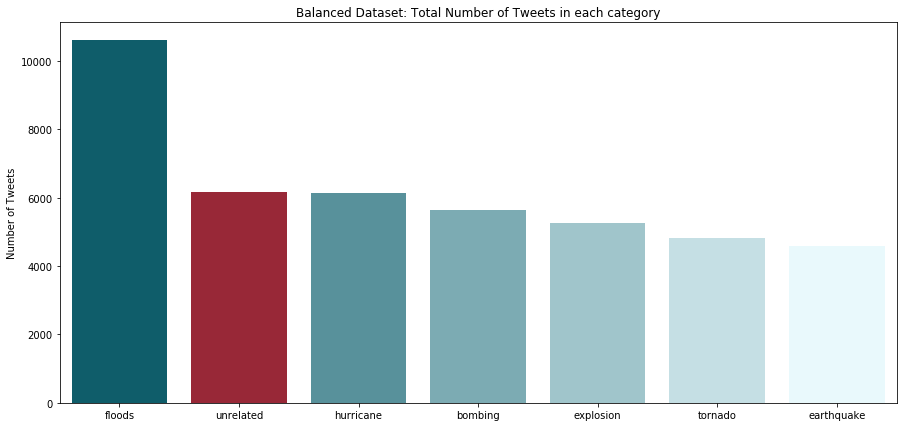## 4. Data Transformation¶

### 4.1 Tokenization¶

One of the common preprocessing task in NLP (Natural Language Processing) is tokenization. Given a character sequence and a defined document unit, tokenization is the task of chopping it up into pieces, called tokens We used the Tokenizer() class from the Keras Preprocessing to vectorize our text data. It will turn our sentences into sequences of integers.We use 10,000 words for this analysis.

We pad all the vectorized text sequences with zeros to make all the sequences of the same length. We use the maximum size to be 100.

In :
class TextTokenizer(BaseEstimator,TransformerMixin):
"""This is a simple Wrapper class for Keras Tokenizer."""
self._num_words=num_words
self.max_length=max_length
self._tokenizer=None
self.vocab_size=None
self.tokenizer=None

def transform(self,X,y=None):
self.tokenizer,self.vocab_size=self._get_tokenizer(X['tweet'])
X['tweet_encoded']=self.tokenizer.texts_to_sequences(X['tweet'])

return X
def _get_tokenizer(self,X):
tokenizer=tf.keras.preprocessing.text.Tokenizer(num_words=self._num_words)
tokenizer.fit_on_texts(X)
vocab_size=len(tokenizer.word_index)+1

In :
tokenization=TextTokenizer(pad_sequences)
dataset_tokenized=tokenization.transform(dataset_resampled)
vocab_size=tokenization.vocab_size
print('Vocab Size:',vocab_size)

Vocab Size: 65246

Out:
tweet label tweet_encoded label_encoded label_one_hot
1 zooduringfloodmtnatstechysonstaffmemberspentwe... floods [7554, 0, 0, 0, 0, 0, 0, 0, 0, 0, 0, 0, 0, 0, ... 3 [0.0, 0.0, 0.0, 1.0, 0.0, 0.0, 0.0]
4 findthelatestlocalfloodinformation:assoutheast... floods [634, 824, 0, 0, 0, 0, 0, 0, 0, 0, 0, 0, 0, 0,... 3 [0.0, 0.0, 0.0, 1.0, 0.0, 0.0, 0.0]
5 floodvictimslookingtogovernmentforhelp-mostins... floods [2366, 7555, 0, 0, 0, 0, 0, 0, 0, 0, 0, 0, 0, ... 3 [0.0, 0.0, 0.0, 1.0, 0.0, 0.0, 0.0]
7 rt911buff::massiveexplosionu/d-localhospitalsn... explosion [41, 1743, 86, 2367, 0, 0, 0, 0, 0, 0, 0, 0, 0... 2 [0.0, 0.0, 1.0, 0.0, 0.0, 0.0, 0.0]
8 caughtoncamera:fertilizerplantexplosionnearwac... explosion [29, 27, 7556, 0, 0, 0, 0, 0, 0, 0, 0, 0, 0, 0... 2 [0.0, 0.0, 1.0, 0.0, 0.0, 0.0, 0.0]

### 4.3 Label Encoding¶

In this step all the target labels are converted to integer values.We use the LabelEncoder class from the Sklean package.

### 4.4 One Hot Encoding¶

In the next step we use the integer values for labels and create a one hot vector to be used for the machine learning analysis.

In :
class LabelOneHotEncoder(BaseEstimator,TransformerMixin):
"""Transfroms the Categorical data to One Hot vector"""
def __init__(self):
self.label_encoder=None
self.one_hot_encoder=None

def transform(self,X,y=None):
self.label_encoder=LabelEncoder().fit(X['label'])
self.one_hot_encoder=to_categorical
num_classes=len(set(X['label']))
X['label_encoded']= self.label_encoder.transform(X['label'].values)
X['label_one_hot']= X['label_encoded'].apply(lambda x: self.one_hot_encoder([x],num_classes=num_classes))

return X

In :
encoder=LabelOneHotEncoder()
dataset_encoded=encoder.transform(dataset_resampled)

Out:
tweet label tweet_encoded label_encoded label_one_hot
1 zooduringfloodmtnatstechysonstaffmemberspentwe... floods [7554, 0, 0, 0, 0, 0, 0, 0, 0, 0, 0, 0, 0, 0, ... 3 [0.0, 0.0, 0.0, 1.0, 0.0, 0.0, 0.0]
4 findthelatestlocalfloodinformation:assoutheast... floods [634, 824, 0, 0, 0, 0, 0, 0, 0, 0, 0, 0, 0, 0,... 3 [0.0, 0.0, 0.0, 1.0, 0.0, 0.0, 0.0]
5 floodvictimslookingtogovernmentforhelp-mostins... floods [2366, 7555, 0, 0, 0, 0, 0, 0, 0, 0, 0, 0, 0, ... 3 [0.0, 0.0, 0.0, 1.0, 0.0, 0.0, 0.0]
7 rt911buff::massiveexplosionu/d-localhospitalsn... explosion [41, 1743, 86, 2367, 0, 0, 0, 0, 0, 0, 0, 0, 0... 2 [0.0, 0.0, 1.0, 0.0, 0.0, 0.0, 0.0]
8 caughtoncamera:fertilizerplantexplosionnearwac... explosion [29, 27, 7556, 0, 0, 0, 0, 0, 0, 0, 0, 0, 0, 0... 2 [0.0, 0.0, 1.0, 0.0, 0.0, 0.0, 0.0]

### 4.3 Word embeddings¶

Word Embedding is a representation of text where words that have the same meaning have a similar representation. In other words it represents words in a coordinate system where related words, based on a corpus of relationships, are placed closer together. In the deep learning frameworks such as TensorFlow, Keras, this part is usually handled by an embedding layer which stores a lookup table to map the words represented by numeric indexes to their dense vector representations.

Word embeddings can be generated using pre-trained word embeddings such as Glove and Word2Vec. Any one of them can be downloaded and used as transfer learning. In this work we use the Embedding Layer of Keras maps the pre-calculated integers to a dense vector of the embedding.

## 5. Train test Data set¶

In this section we split our data into training and testing datasets.It is important to use a splitting strategy that preserve the percentage of samples for each class.We use the train_test_split tool from the sklean library to achieve this goal.

In :
X_train,X_test,y_train,y_test =train_test_split(dataset_encoded['tweet_encoded'],dataset_encoded['label_one_hot'],test_size=0.3,stratify=dataset_encoded['label_encoded'])
X_train=np.array(X_train.values.tolist())
X_test=np.array(X_test.values.tolist())
y_train=np.array(y_train.values.tolist())
y_test=np.array(y_test.values.tolist())
print('Number of Tweets in Training set: ',X_train.shape)
print('Number of Tweets in Test set: ',X_test.shape)

Number of Tweets in Training set:  30250
Number of Tweets in Test set:  12965


## 5. Modeling¶

### 5.1 Model Architecture¶

For the modeling we will use the Keras's Sequential model API.The Sequential model is essentially a linear stack of layers.We can use different types of available Keras layers in this model.

In :
max_length=100
embeding_dim=50
num_classes=y_train.shape
model=Sequential()
model.summary()

Model: "sequential_1"
_________________________________________________________________
Layer (type)                 Output Shape              Param #
=================================================================
embedding (Embedding)        (None, 100, 50)           3262300
_________________________________________________________________
global_max_pooling1d (Global (None, 50)                0
_________________________________________________________________
dropout (Dropout)            (None, 50)                0
_________________________________________________________________
dense (Dense)                (None, 10)                510
_________________________________________________________________
dropout_1 (Dropout)          (None, 10)                0
_________________________________________________________________
dense_1 (Dense)              (None, 7)                 77
=================================================================
Total params: 3,262,887
Trainable params: 3,262,887
Non-trainable params: 0
_________________________________________________________________

In :
class PlotLosses(tf.keras.callbacks.Callback):
"""Simple utility function to plot the model losses during training"""
def on_train_begin(self, logs={}):
self.i = 0
self.x = []
self.losses = []
self.val_losses = []

self.fig = plt.figure()

self.logs = []

def on_epoch_end(self, epoch, logs={}):

self.logs.append(logs)
self.x.append(self.i)
self.losses.append(logs.get('loss'))
self.val_losses.append(logs.get('val_loss'))
self.i += 1

clear_output(wait=True)
plt.plot(self.x, self.losses, label="loss")
plt.plot(self.x, self.val_losses, label="val_loss")
plt.legend()
plt.show();
plot_losses = PlotLosses()

def save_model(model,save_name):
with open(save_name,'w+') as f:
f.write(model.to_json())
model.save_weights(save_name+'.h5')


### 5.2 Training¶

In [ ]:
model.fit(X_train,y_train,epochs=2,batch_size=10,verbose=0,validation_data=(X_test,y_test),callbacks=[plot_losses])
save_model(model,'model')


### 5.3 Evaluation¶

In [ ]:
# load json and create model
json_file = open('model', 'r')
json_file.close()
# load weights into new model

# evaluate loaded model on test data


### 5.5 Hyperparameters Optimization¶

In :
def create_model(dropout, dense_size, vocab_size, embedding_dim, maxlen):
model=Sequential()
return model

In [ ]:
# Main settings
epochs = 5
embedding_dim = 50
maxlen = 100
vocab_size=10000
output_file = 'output.txt'
dense_size=[10, 50,100],
# Parameter grid for grid search
param_grid = dict(dropout=[0.1],
dense_size=[10, 50,100],
vocab_size=[vocab_size],
embedding_dim=[embedding_dim],
maxlen=[maxlen])
model = KerasClassifier(build_fn=create_model,
epochs=epochs, batch_size=10,
verbose=False)
grid = RandomizedSearchCV(estimator=model, param_distributions=param_grid,
cv=4, verbose=1, n_iter=5 ,n_jobs=2)
grid_result = grid.fit(X_train, y_train)

# Evaluate testing set
test_accuracy = grid.score(X_test, y_test)
# Save and evaluate results
with open(output_file, 'a') as f:
s = ('Best Accuracy : '
'{:.4f}\n{}\nTest Accuracy : {:.4f}\n\n')
output_string = s.format(
grid_result.best_score_,
grid_result.best_params_,
test_accuracy)
print(output_string)
f.write(output_string)
print('Done')